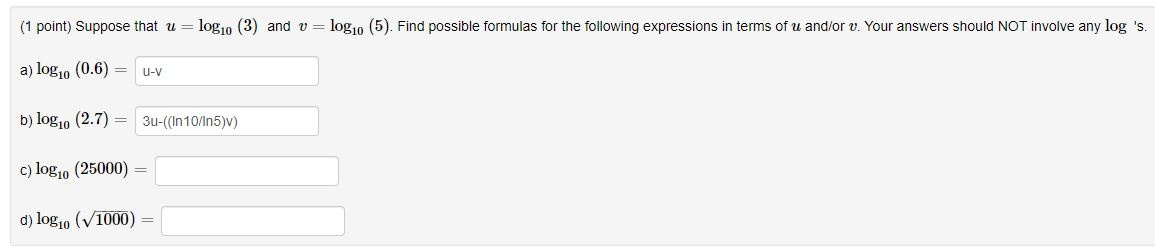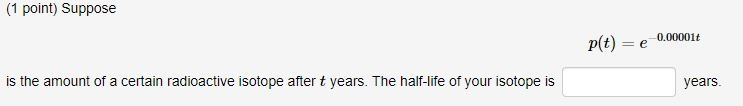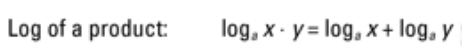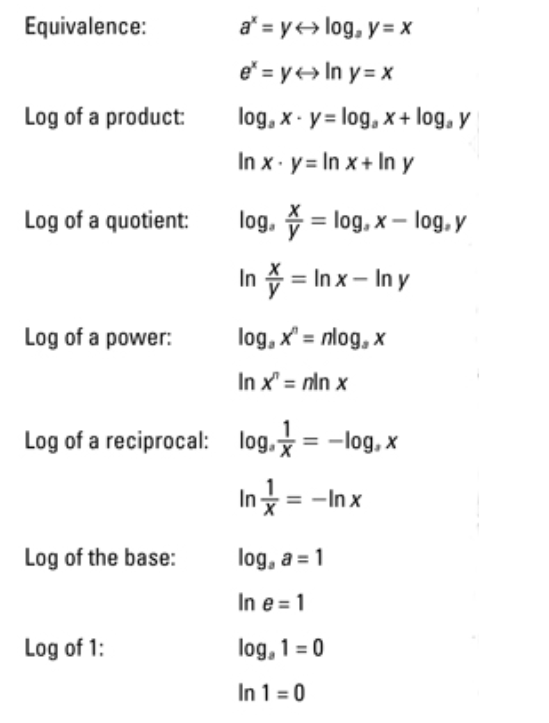## When Helping Means Winning contest

Ask a school-related question in the Help Zone and you could win one of three \$500 scholarships.

# Help Zone

### Student QuestionFor the problem on top, I don't know how to do c and d. For the one down, I do not know how to even begin.

Mathematics## Explanations (1)

•Explanation from AlloprofExplanation from Alloprof

This Explanation was submitted by a member of the Alloprof team.

Hi !

Let's begin with c :

25000 can be written as 5*5*1000

So log(25000) = log (5*5*1000)

Now you can use one of the laws of logs. In this exercise will use :So log (5*5*1000) = log(5) + log(5) + log(1000)

Well, we know that log(1000) = 3

So we can rewrite our equation :

log (25000) = 2*log(5) + 3 = 2*v+3

I did all the c with you, but for the d I ll just give you some tips.

tip 1 : rewrite sqrt(1000) as 1000^1/2

tip 2 : use one of the laws of logs----------------------------------------------------------------

You have to know that half-life of an isotope means the time taken for the radioactivity of a specified isotope to fall to half its original value.

You have the function :

P(t) = e^(-0.00001*t) and I suppose that p(t) is a proportion.

You have to find the time taken of your isotope to fall to half its original value, so 50%

In other words, you have to isolate t in : P(t) = e^(-0.00001*t)

Have a nice day

KH